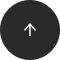PostgreSQL

# How to Get a Remainder Using MOD() in PostgreSQL, MS SQL Server, and MySQL

#### Operators:

MOD, ABS, CASE WHEN, SIGN

#### Problem:

You want to find the (non-negative) remainder.

#### Example:

In the table `numbers`, you have two columns of integers: `a` and `b`.

ab
93
53
23
03
-23
-53
-93
5-3
-5-3
50
00

You want to compute the remainders from dividing `a` by `b`. Each remainder should be a non-negative integer value smaller than `b`.

#### Solution 1 (not entirely correct):

```SELECT
a,
b,
MOD(a, b) AS remainder
FROM numbers;
```

The result is:

abremainder
930
532
232
030
-23-2
-53-2
-930
5-32
-5-3-2
50error
00error

#### Discussion:

This solution works correctly if a is non-negative. However, when it is negative, it doesn’t follow the mathematical definition of the remainder.

Conceptually, a remainder is what remains after an integer division of ```a ``` by `b`. Mathematically, a remainder of two integers is a non-negative integer that is smaller than the divisor `b`. More precisely, it is a number r∈{0,1,...,b - 1} for which there exists some integer k such that a = k * b + r. E.g.:

`5 = 1 * 3 + 2`, so the remainder of 5 and 3 equals `2`.

`9 = 3 * 3 + 0`, so the remainder of 9 and 3 equals `0`.

`5 = (-1) * (-3) + 2`, so the remainder of 5 and -3 equals `2`.

This is how `MOD(a, b)` works for the non-negative dividends in the column `a`. Obviously, an error is shown if the divisor `b` is `0`, because you can't divide by `0`.

Getting the correct remainder is problematic when the dividend a is a negative number. Unfortunately, `MOD(a, b)` can return a negative value when a is negative. E.g.:

`MOD(-2, 5)` returns `-2` when it should return `3`.

`MOD(-5, -3)` returns `-2` when it should return `1`.

#### Solution 2 (correct for all numbers):

```SELECT
a,
b,
CASE WHEN MOD(a, b) >= 0
THEN MOD(a, b)
ELSE
MOD(a, b) + ABS(b)
END AS remainder
FROM numbers;
```

The result is:

abremainder
930
532
232
030
-231
-531
-930
5-32
-5-31
50error
00error

#### Discussion:

To compute the remainder of a division between any two integers (negative or non-negative), you can use the `CASE WHEN` construction. When `MOD(a, b)` is non-negative, the remainder is simply `MOD(a, b)`. Otherwise, we have to correct the result returned by `MOD(a, b)`.

How do you get the correct remainder when `MOD()` returns a negative value? You should add the absolute value of the divisor to `MOD(a, b)`. That is, make it `MOD(a, b) + ABS(b)`:

`MOD(-2, 5)` returns `-2` when it should return `3`. You can fix this by adding `5`.

`MOD(-5, -3)` returns `-2` when it should return `1`. You can fix this by adding `3`.

When `MOD(a, b)` returns a negative number, the `CASE WHEN` result should be `MOD(a, b) + ABS(b)`. This is how we get Solution 2. If you need a refresher on how the `ABS()` function works, take a look at the cookbook How to compute an absolute value in SQL.

Of course, you still can't divide any number by `0`. So, if `b = 0`, you'll get an error.

#### Solution 3 (correct for all numbers):

```SELECT
a,
b,
MOD(a, b) + ABS(b) * (1 - SIGN(MOD(a, b) + 0.5)) / 2 AS remainder
FROM numbers;
```

The result is:

abremainder
930
532
232
030
-231
-531
-930
5-32
-5-31
50error
00error

#### Discussion:

There is another way to solve this problem. Instead of a `CASE WHEN`, use a more complex one-line mathematical formula:

`MOD(a, b) + ABS(b) * (1 - SIGN(MOD(a, b) + 0.5)) / 2`

In Solution 2, `MOD(a, b) + ABS(b)` was returned for cases when `MOD(a, b) < 0`. Note that `MOD(a, b) + ABS(b) = MOD(a, b) + ABS(b) * 1 when MOD(a, b) < 0`.

In contrast, you return `MOD(a, b)` when `MOD(a, b) >= 0`. Note that `MOD(a, b) = MOD(a, b) + ABS(b) * 0 when MOD(a, b) >= 0`.

So, we can multiply `ABS(b)` by an expression that equals 1 for a negative `MOD(a, b)` and `0` for a non-negative `MOD(a, b)`. Since `MOD(a, b)` is always an integer, the expression `MOD(a, b) + 0.5` is always positive for `MOD(a, b) ≥ 0` and negative for `MOD(a, b) < 0`. You can use any positive number less than `1` instead of `0.5`.

The sign function `SIGN()` returns `1` if its argument is strictly positive, `-1` if it is strictly negative, and `0` if it equals `0`. However, you need something that returns only `0` and `1`, not `1` and `-1`. Here is how you fix this:

`(1 - 1) / 2 = 0`

`(1 - (-1)) / 2 = 1`

Then, the correct expression by which you multiply `ABS(b)` is:

`(1 - SIGN(MOD(a, b) + 0.5)) / 2`

So, the entire formula is:

`MOD(a, b) + ABS(b) * (1 - SIGN(MOD(a, b) + 0.5)) / 2`

#### Recommended articles: